Machine Learning: A probabilistic perspective

## Logistic Regression

• 生成模型，建模$p(x, y)$，然后利用贝叶斯公式得到后验概率$p(y|x)$.
• 判别模型，直接建模$p(y|x)$.

### 模型拟合

$$NLL(w) = - \sum_{i=1}^N \left[ y_i \log \mu_i (1-y_i) \log (1-\mu_i) \right]$$

$$NLL(w) = - \sum_{i=1}^N \log(1+\exp(y_i w^T x))$$

closed form: 由常数，变量，通过四则运算，n次方，指数，对数，三角函数，反三角函数，经过有限次运算和符合得到的表达式（通常没有极限运算）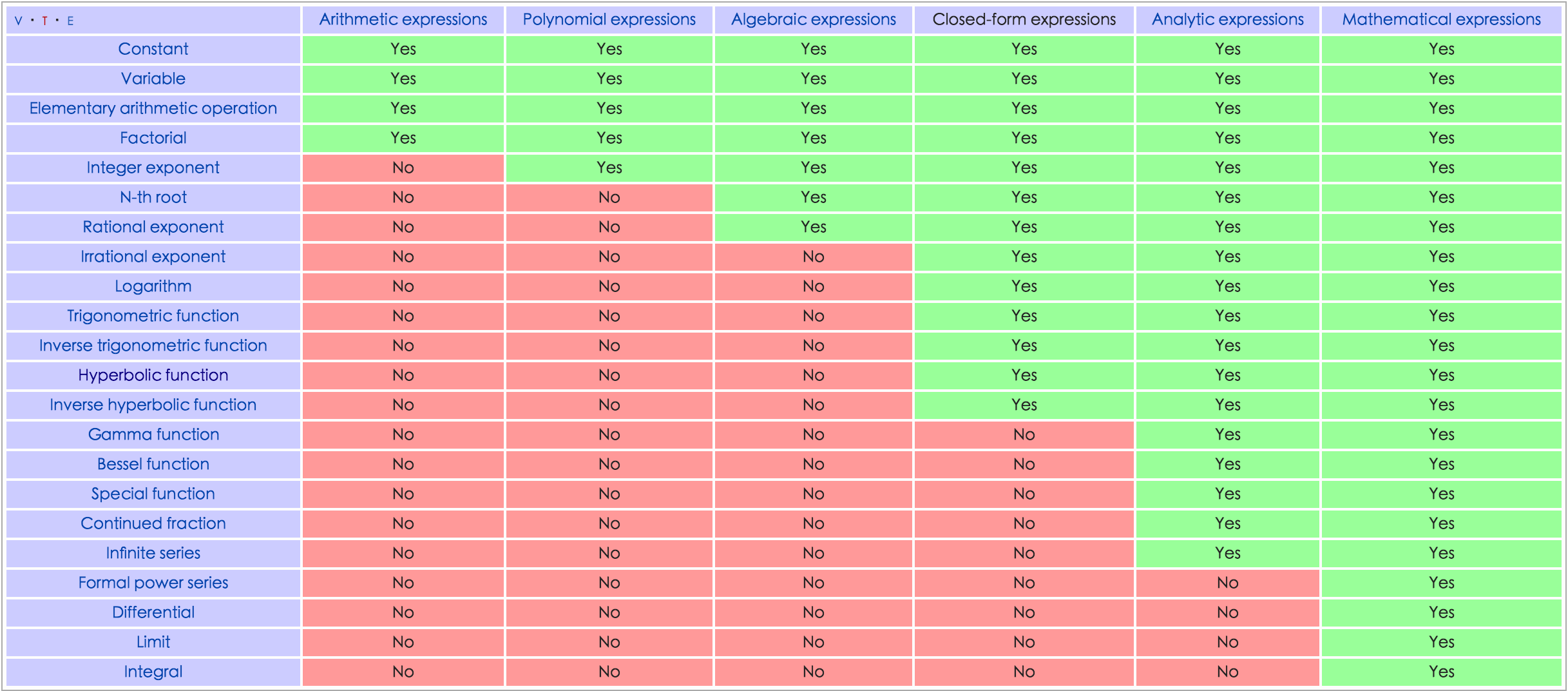NLL 无法表达为闭形式？所以，采用数值优化，需要计算梯度和海森矩阵！

$$g = X^T (\mu - y) \\ H = \sum_i \mu_i (1-\mu_i) x_i x_i^T = X S X^T$$

H 是正定的，所以NLL是强凸函数，且存在唯一解。

$\eta_k = \arg \min_{\eta>0} f(\theta + \eta d)$ d是下降的负梯度方向。

$$\theta_{k+1} = \theta_k - \eta_k g_k + \mu_k (\theta_k - \theta_{k-1}) \\ 0 \le \mu_k \le 1$$

iteratively reweighted least squares or IRLS 优化 for 逻辑回归。

$$w_{k+1} = w_k - H^{-1}g_k \\ = (X^T S_k X)^{-1} X^T S_k z_k \\ z_k = Xw_k + S_k^{-1}(y-\mu_k)$$

MAP 估计导致正则项，高斯先验：l2正则。

### Bayesian logistic regression

$$p(\theta| \mathcal{D}) = \frac{1}{Z} e^{-E(\theta)}$$

$E(\theta)$ 叫做能量函数，等于$- p(\theta, \mathcal{D})$，而$Z = p(\mathcal{D})$。

$$E(\theta) \approx E(\theta*) + (\theta - \theta*)^T g + \frac{1}{2} (\theta - \theta*)^T H (\theta - \theta*)$$

g是能量函数在最低能量位置的梯度，等于0，所以

$$\hat{p}(\theta| \mathcal{D}) \approx \frac{1}{Z} e^{-E(\theta*)} \exp \left[ -\frac{1}{2} (\theta - \theta*)^T H (\theta - \theta*) \right] \\ = \mathcal{N}(\theta | \theta* , H^{-1}) \\ Z = p(\mathcal{D}) \approx e^{-E(\theta*)} (2\pi)^{D/2} |H|^{-1/2}$$

### Drivation of BIC

$$p(\mathcal{D}) \approx \log p(\mathcal{D}|\theta^* ) + \log p(\theta^*) - \frac{1}{2} \log |H|$$

$p(\mathcal{D}|\theta^* )$ 常称作 Occam factor，用作模型复杂度的度量，如果假定均匀分布先验，即$p(\theta^) \varpropto 1$，那么可以简化为极大似然估计，可以用MLE的值$\hat{\theta}$替换$\theta^*$。

$$\log |H| = \log |N \hat{H}| = \log (N^D \hat{H}) \\ = D \log N + \log |\hat{H}|$$

$$\log p(\mathcal{D}) = \log p(\mathcal{D}|\hat{\theta}) - \frac{D}{2} \log N$$

### 高斯近似

$$p(w | \mathcal{D}) \approx \mathcal{N}(w|\hat{w}, H^{-1})$$

### 后验预测

$$p(y| x, \mathcal{D}) = \int p(y|x,w) p(w| \mathcal{D}) dw$$

$$p(y| x, \mathcal{D}) \approx p(y| x, \mathbb{E}[w])$$

$\mathbb{E}[w]$称作贝叶斯点！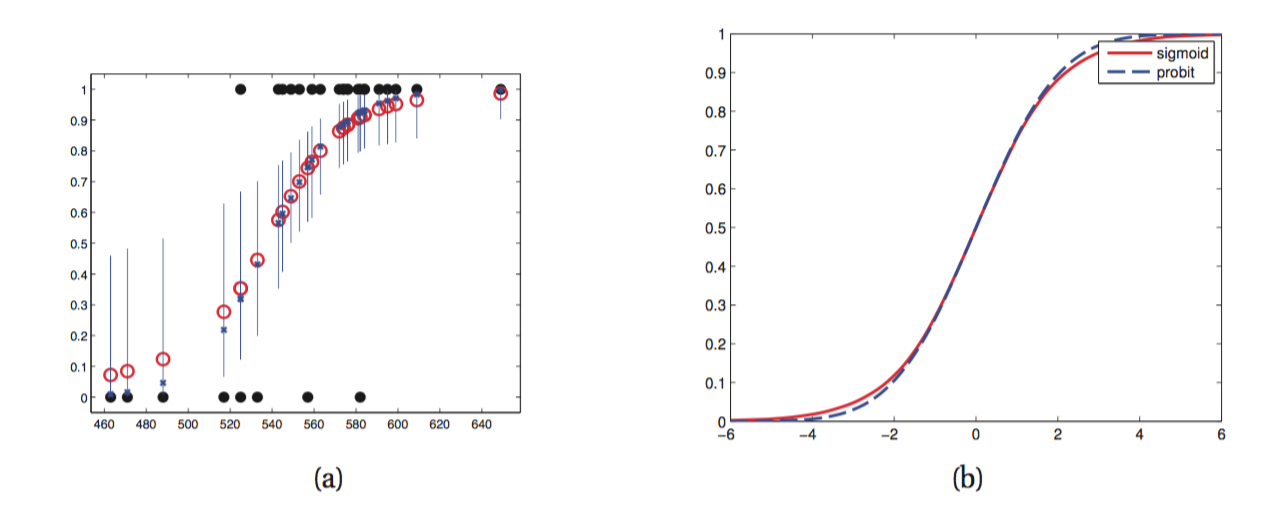$$p(y=1| x, \mathcal{D}) \approx \frac{1}{S} \sum_{s=1}^S sigm((w^s)^T x)$$

$w^s \sim p(w|\mathcal{D})$ 采样自后验分布！高斯近似下，就相当于采样高斯分布！

probit 近似 当w的后验分布用高斯近似$\mathcal{N}(w|m_N, V_N)$时，可以

$$p(y=1| x, \mathcal{D}) \approx \int sigm(w^T x) p(w|\mathcal{D}) dw \\ = \int sigm(a) \mathcal{N}(a|\mu_a, \sigma_a^2) \\ a = w^T x \\ \mu_a = \mathbb{E}[a] = m_N^T x \\ \sigma_a^2 = x^T V_N x.$$

$$\int sigm(a) \mathcal{N}(a|\mu_a, \sigma_a^2) \approx sigm(k(\sigma^2)\mu) \\ k(\sigma^2) = (1+\pi \sigma^2/8)^{-\frac{1}{2}}.$$

Residul analysis(outlier detection)

## 广义线性模型和指数族 GLM

### 指数族分布

#### 重要性：

• finite-sized sucient statistics.

under certain regularity conditions, the exponential family is the only family of distributions with finite-sized sucient statistics, meaning that we can compress the data into a fixed-sized summary without loss of information. This is particularly useful for online learning, as we will see later.

• 唯一存在共轭先验的分布族

• least set of assumptions

The exponential family can be shown to be the family of distributions that makes the least set of assumptions subject to some user-chosen constraint

#### 定义

$$p(x) = h(x) \exp(\theta^T \phi(x) - A(\theta)) \\ = \frac{1}{Z(\theta)}h(x) e^{\theta^T \phi(x)}$$

$Z$是归一化因子，$A$是对数归一化因子，$\theta$被称作自然参数。
$\phi(x) \in \mathcal{R}^d$被称为充分统计向量(vector of sucient statistics)。

Log partition function 性质：

• $A(\theta)$的一阶导是$\phi(x)$的期望，而二阶导是其协方差矩阵！即对数矩母函数！

#### 极大似然估计

N个iid的样本的对数似然函数为：

$$l(\theta) = \theta^T \sum_i \phi(x_i) - N A(\theta) + constant$$

$$\nabla_{\theta} l = 0 \\ \nabla_{\theta} A = E \phi(X)$$

$$E \phi(X) = \frac{1}{N} \sum_i \phi(x_i)$$

Pitman-Koopman-Darmois theorem 理论：在一些约束下，指数族是为一个分布族，其充分统计量是有限的（不随样本个数增加而增加）！

#### 贝叶斯统计

$$p(\mathcal{D}|\theta) \varpropto g(\theta)^N \exp(\eta(\theta)^T s_N)$$

$$p(\mathcal{D}|\eta) \varpropto g(\eta)^N \exp(N \eta^T \bar{s} - N A(\eta))$$

$$p(\eta|v_0, \bar{\tau_0}) \varpropto g(\eta)^N \exp(v_0 \eta^T \bar{\tau_0} - v_0 A(\eta))$$

$$p(\theta|\mathcal{D}) = p(\theta| v_0 +N , \tau_0 + s_N) \\ p(\eta|\mathcal{D}) = p(\eta| v_0 +N , \frac{v_0 \bar{\tau_0} + N \bar{s}}{v_0 + N})$$

#### 最大熵原理

$$\max -\sum_x p(x) \log(p(x)) \\ s.t. \sum_x f_k(x)p(x) = F_k, k=1,...,m \\ \sum_x p(x)=1$$

$$p(x) = \frac{1}{Z} \exp(\lambda_k f_k(x))$$

### 广义线性模型 GLM

GLM：均值函数是输入的线性组合，然后加上一个非线性变换！输出是指数族分布的模型！

$$p(y_i| \theta, \sigma^2) = \exp \left[\frac{y_i \theta - A(\theta)}{\sigma^2} + c(y_i, \sigma^2) \right]$$

$\theta$是自然参数，$\sigma$是dispersion parameter（通常是1），A是 partition function 和指数族里面的 A 一样！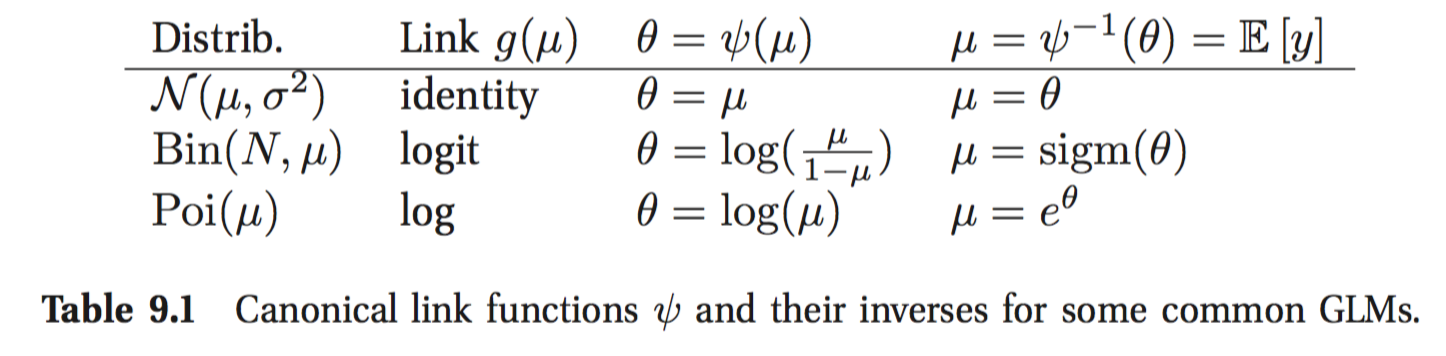$$\mu_i = g^{-1}(\eta_i) = g^{-1}(w^T x_i)$$

$$p(y_i| \theta, w, \sigma^2) = \exp \left[\frac{y_i w^T x_i - A(w^T x_i)}{\sigma^2} + c(y_i, \sigma^2) \right]$$

• 线性回归，$y_i \in \mathbb{R}, \theta_i = \mu_i = w^T x_i, A(\theta)=\theta^2/2$
• binomial回归，$y_i\in{0,1,...,N_i}, \pi_i = sigm(w^T x_i), \theta = \log(\pi_i / (1-\pi_i)) = w^T x$
• 泊松回归，$y_i \in \mathcal{N}^+, \mu_i = exp(\theta), \theta=w^T x$

$$\mathbb{E}[y|x,w,\sigma^2] = \mu_i = A'(\theta) \\ Var[y|x,w,\sigma^2] = \sigma_i^2 = \sigma^2 A''(\theta) \\$$

$$l(w) = \log p(\mathcal{D}|w) = \frac{1}{\sigma^2}\sum_{i=1}^N l_i \\ l_i = \theta_i y_i - A(\theta_i)$$

$$\nabla_w l = \frac{1}{\sigma^2} \sum_{i=1}^N (y_i - \mu_i) x_i$$

$$H = X^T S X \\ S = diag\{... \frac{d\mu_i}{d\theta_i} ...\}$$

Fisher scoring method?

### Probit regression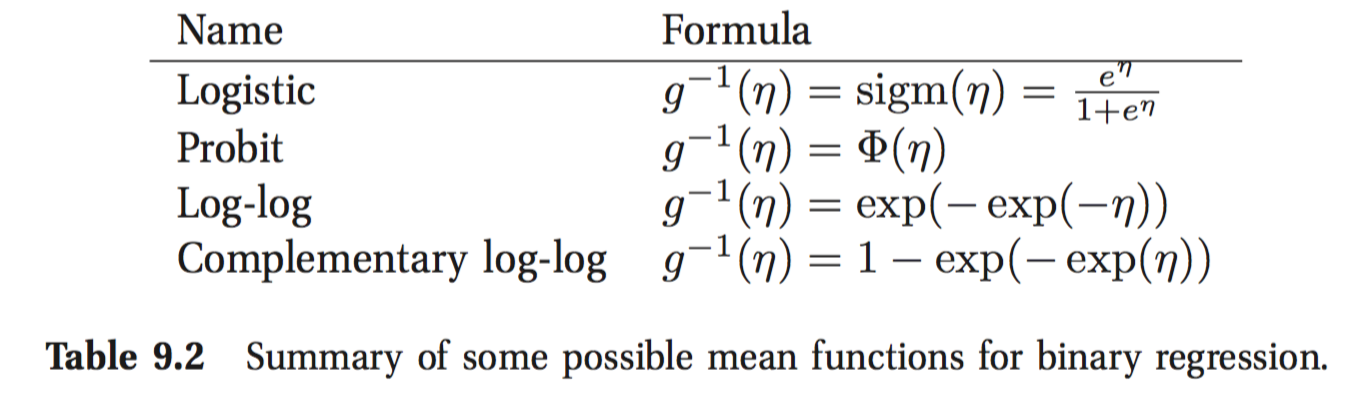$$u_{0,i} = w_0 ^T x_i + \delta_{0,i} \\ u_{1,i} = w_1 ^T x_i + \delta_{1,i} \\ y_i = \mathbb{I}(u_{1,i} > u_{0,i})$$

$$p(y_i=1|x_i, w) = \Phi(w^T x_i)$$

Multinomial probit models：直接看模型数学公式

$$z_{ic} = w^T x_{ic} + \epsilon_{ic} \\ \epsilon \sim \mathcal{N}(0, R) \\ y_i = \arg \max_c z_{ic}$$

• transfer learning (e.g., (Raina et al. 2005))
• learning to learn (Thrun and Pratt 1997)

$$\mathbb{E}[y_i | x_i, u=j] = (\beta_*^T + w_j)^T x_i$$

### Learn to rank

$$sim(q, d) = p(q | d) = \Pi_{i=1}^n p(q|q_i)$$

The pointwise approach: 对每一个q和文档d，生成一个特征向量$x(q, d)$，

pairwise： 学习 $p(y_{jk}=1| x(q, d_j), x(q, d_k))$，$y_{jk}=1$表示文档j相关度大于文档k相关度，

$$p(y_{jk}=1| x(q, d_j), x(q, d_k)) = sigm(x(q, d_j) - x(q, d_k))$$

The listwise approach：学习一个排列$\pi$。分布

$$p(\pi|s) = \Pi_{j=1}^m \frac{s_j}{\sum_{u=j}^m s_n}$$

$s_j = s(\pi^{-1}(j))$ 是文档排列在第j个位置的时候的score！

$$p(\pi|s) = \frac{s_A}{s_A+s_B+s_C} \times \frac{s_B}{s_B+s_C} \times \frac{s_C}{s_C}$$

ListNet Cao et al. 2007

## Latent linear models

### 因子分析

$$p(z_i) = \mathcal{N}(z_i | \mu_0, \Sigma_0)$$

$$p(x_i | z_i, \theta) = \mathbb{N}(W z_i + \mu, \Phi)$$

W 被称为 factor loading matrix， 而应变量称为因子，被强制要求为能够解释观测变量之间的相关性，此时$\Phi$是对角的！

• 因子分析作为协方差的低秩矩阵分解

$$p(x_i | \theta) = \mathbb{N}(x_i | W\mu_0 + \mu,\Phi + W\Sigma_0 W^T)$$

$$\text{Cov}(x) = W W^T + \Phi$$

$$p(z_i| x_i, \theta) = \mathcal{N}(z_i | m_i, \Sigma_i) \\ \Sigma_i = (\Sigma_0 + W^T \Phi^{-1} W)^{-1} \\ m_i = \Sigma_i(W^T \Phi^{-1} (x_i - \mu) + \Sigma_0^{-1} \mu_0)$$

m 被称作隐因子 or latent score.

W不是唯一的！！ 例如可以通过做一个正交变换R，RW 仍然是有效的！解决方法，增加约束。

• 要求W是正交的，然后按照 latent factor 的方差排序： PCA
• 要求W是下三角，即每个观测变量只与前面的因子有关
• 稀疏约束：l1 regularization (Zou et al. 2006), ARD (Bishop 1999; Archambeau and Bach 2008), or spike-and-slab priors (Rattray et al. 2009).
• Choosing an informative rotation matrix. varimax
• 非高斯先验 for 隐变量：ICA

### 混合因子分析 (Hinton et al. 1997)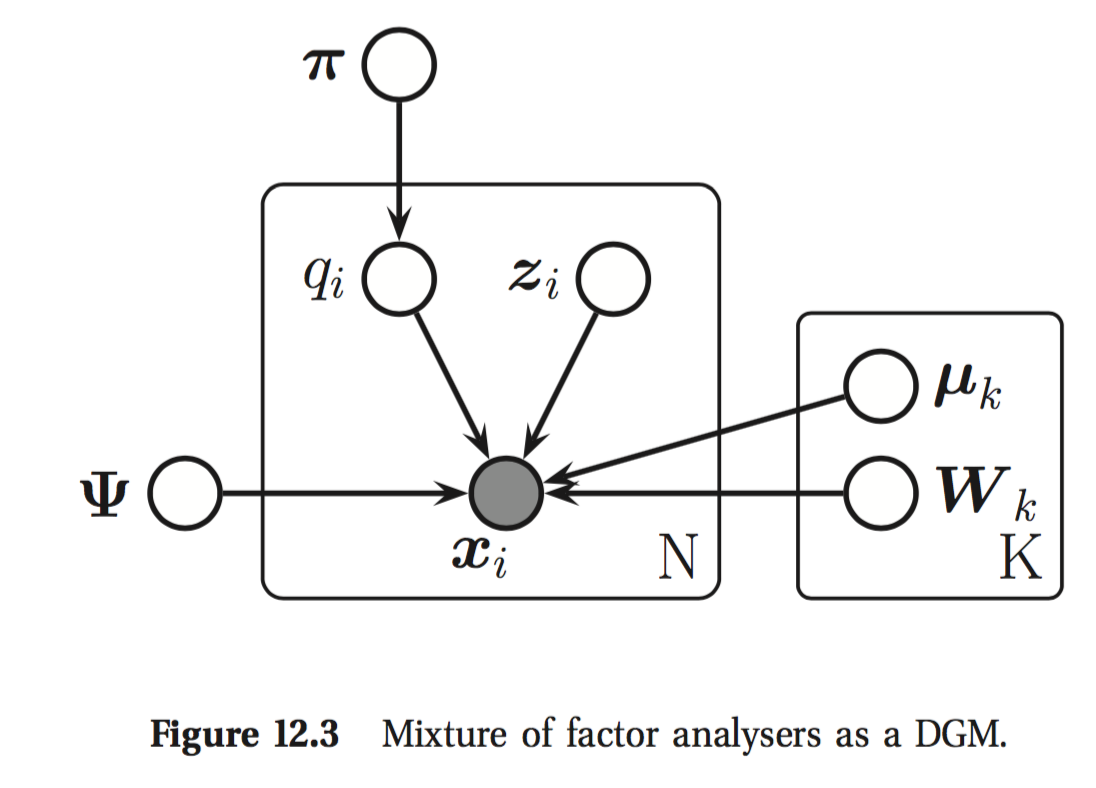$$p(x_i| z_i, q_i = k, \theta) = \mathcal{N}(x_i | \mu_k + W_k z_i, \Phi) \\ p(z_i| \theta) = \mathcal{N}(z_i| 0, I) \\ p(q_i| \theta) = \text{Cat}(q_i| \pi)$$

### 因子分析的EM算法

$$r_{ic} = p(q_i=c| x_i, \theta) \propto \pi_{c} \mathcal{N}(x_i|\mu_c, W_cW_c^T + \Phi) \\$$

## PCA

(Tipping and Bishop 1999): 当 $\Phi = \sigma^2 I$ 并且 W 矩阵是正交的，随着$\sigma^2 \rightarrow 0$，

### Classical PCA

$$\min J(W, Z) = \sum_{i=1}^N || x_i - \hat{x_i}||^2 = || X - WZ^T ||^2_F \\ s.t. W^T W = I_L .$$

F表示Frobenius范数。

### PPCA (Tipping and Bishop 1999)

$$\log p(X| W, \sigma^2) &= - \frac{N}{2} \log |C| - \frac{1}{2} \sum_{i=1}^N x_i^T C^{-1} x_i \\ &= - \frac{N}{2} \log |C| - \frac{1}{2}$$